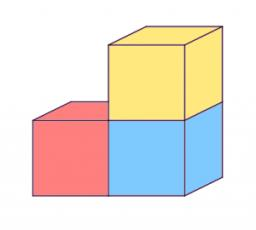# Decreases 5625

How much percent will the surface and volume of the cube decrease if the diagonal decreases by 10%?
b) if the diagonal increases by 10%?

p1 =  19 %
p2 =  27.1 %
p3 =  21 %
p4 =  33.1 %

### Step-by-step explanation:Did you find an error or inaccuracy? Feel free to write us. Thank you!

Tips for related online calculators